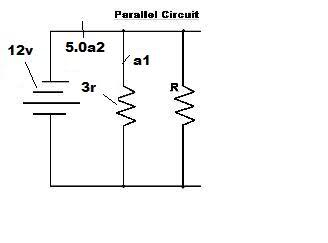# Determine the equivalent resistance of the circuit

• zelda1850
So the resistance of the unknown resistor is 12 Ohms. In summary, the given conversation discusses a circuit with a 3.0 ohm resistor, an unknown resistor r, and two ammeters a1 and a2, connected with a 12 volt source. Ammeter a2 reads a current of 5.0 amperes. The equivalent resistance of the circuit is found to be 2.4 ohms and the current measure of ammeter a1 is calculated to be 4 amperes. Using the formula 1/req = 1/r1 + 1/r2, the resistance of the unknown resistor is determined to be 12 ohms.

## Homework Statement

a 3.0 ohm resistor an unkown resistor r and two ammeters a1 and a2 are connected with a 12 volt source ammeter a2 reads a current of 5.0 amperes

1) detemine the equivalent resistance of the circuit
2) caculate the current measure of ammeter a 1
3) caculate the resistance of the unknown resistor r

## Homework Equations

v= 12 v
a2 = 5.0 a
a1 = ?
r1 = 3.0 ohm
r2 =?

this is a parallel circuit so

do i use v/a to find equivalent resistance?

for current do i use v/r to find a?

and for the resistor is this equation right 1/req = 1/r1 + 1/r2

## The Attempt at a Solution

i got 2.4 ohm for the equivalent resistance and 4 a for the current don't know if it right

There's no way to answer this without seeing the circuit.

oh here a picture of the diagram for the problemThe answers you have so far are correct. What exactly makes you doubt your solution?

You are correct so far, so why not go on to find the resistance of the unknown resistor using the formula you have: $$\frac {1}{R_{eq}} = \frac{1}{R_{1}} + \frac{1}{R_{2}}$$

ok i got 12 r for the resistor using that equation

zelda1850 said:
ok i got 12 r for the resistor using that equation

Which is correct, apart from the units; the units of resistance are Ohms, $$\Omega$$.